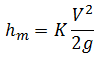Minor Loss Calculation for Liquids and Gases

Minor loss due to fittings in pipes and open channels

 Select units: Use feet and seconds units Use meters and seconds units Minor Loss Coefficient, K: © 2014 LMNO Engineering, Velocity, V (ft/s): Research, and Software, Ltd. Minor Loss, hm (ft): http://www.LMNOeng.com

Units in minor loss calculator: ft=foot, m=meter, s=second.

Minor Loss Equation:g = acceleration due to gravity = 32.174 ft/s2 = 9.806 m/s2.

hm = head loss due to a fitting and has units of ft or m of fluid. It is the energy loss due to a fitting per unit weight of fluid.

K = minor loss coefficient for valves, bends, tees, and other fittings - table of minor loss coefficients.

The minor loss calculation is valid for open channels (including partially full culverts) as well as closed conduits (circular or non-circular) flowing full. The minor loss calculation does not check for unreasonable inputs such as negative values. All values should be entered as positive.

© 1998-2015 LMNO Engineering, Research, and Software, Ltd.  All rights reserved.

LMNO Engineering, Research, and Software, Ltd.
7860 Angel Ridge Rd.   Athens, Ohio 45701  USA   Phone: (740) 592-1890
LMNO@LMNOeng.com    http://www.LMNOeng.com

To:
LMNO Engineering home page (more calculations)

Minor Loss Coefficients (K)

Q=VA simple flowrate calculation

Unit Conversions

Other information:

Register

Discussion and References